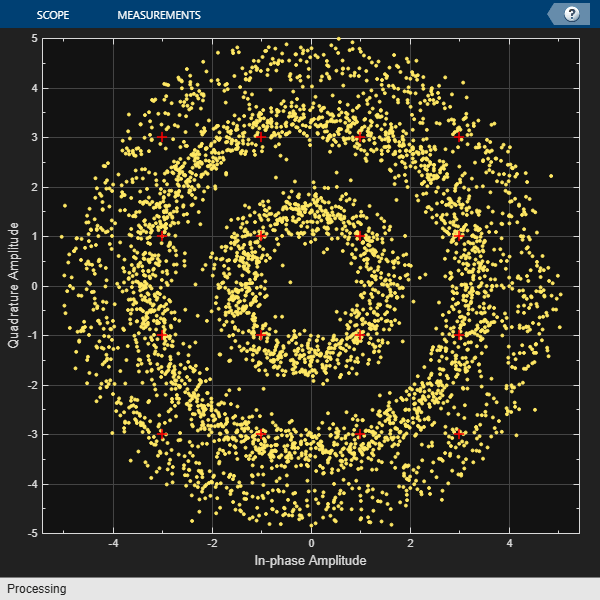## Compensate for Frequency Offset Using Coarse and Fine Compensation

Correct for a phase and frequency offset in a noisy QAM signal using a carrier synchronizer. Then correct for the offsets using both a carrier synchronizer and a coarse frequency compensator.

Set the example parameters.

```fs = 10000; % Symbol rate (Hz) sps = 4; % Samples per symbol M = 16; % Modulation order k = log2(M); % Bits per symbol ```

Create a QAM modulator and an AWGN channel.

```channel = comm.AWGNChannel('EbNo',20,'BitsPerSymbol',k,'SamplesPerSymbol',sps); ```

Create a constellation diagram object to visualize the effects of the offset compensation techniques. Specify the constellation diagram to display only the last 4000 samples.

```constdiagram = comm.ConstellationDiagram(... 'ReferenceConstellation',qammod(0:M-1,M), ... 'SamplesPerSymbol',sps, ... 'SymbolsToDisplaySource','Property','SymbolsToDisplay',4000, ... 'XLimits',[-5 5],'YLimits',[-5 5]); ```

Introduce a frequency offset of 400 Hz and a phase offset of 30 degrees.

```phaseFreqOffset = comm.PhaseFrequencyOffset(... 'FrequencyOffset',400,... 'PhaseOffset',30,... 'SampleRate',fs); ```

Generate random data symbols and apply 16-QAM modulation.

```data = randi([0 M-1],10000,1); modSig = qammod(data,M); ```

Create a raised cosine filter object and filter the modulated signal.

```txfilter = comm.RaisedCosineTransmitFilter('OutputSamplesPerSymbol',sps, ... 'Gain',sqrt(sps)); txSig = txfilter(modSig); ```

Apply the phase and frequency offset, and then pass the signal through the AWGN channel.

```freqOffsetSig = phaseFreqOffset(txSig); rxSig = channel(freqOffsetSig); ```

Apply fine frequency correction to the signal by using the carrier synchronizer.

```fineSync = comm.CarrierSynchronizer('DampingFactor',0.7, ... 'NormalizedLoopBandwidth',0.005, ... 'SamplesPerSymbol',sps, ... 'Modulation','QAM'); rxData = fineSync(rxSig); ```

Display the constellation diagram of the last 4000 symbols.

```constdiagram(rxData) ```Even with time to converge, the spiral nature of the plot shows that the carrier synchronizer has not yet compensated for the large frequency offset. The 400 Hz offset is 1% of the sample rate.

Repeat the process with a coarse frequency compensator inserted before the carrier synchronizer.

Create a coarse frequency compensator to reduce the frequency offset to a manageable level.

```coarseSync = comm.CoarseFrequencyCompensator('Modulation','QAM','FrequencyResolution',1,'SampleRate',fs*sps); ```

Pass the received signal to the coarse frequency compensator and then to the carrier synchronizer.

```syncCoarse = coarseSync(rxSig); rxData = fineSync(syncCoarse); ```

Plot the constellation diagram of the signal after coarse and fine frequency compensation.

```constdiagram(rxData) ```The received data now aligns with the reference constellation.